# Ch-2 AC.pdf

31 May 2023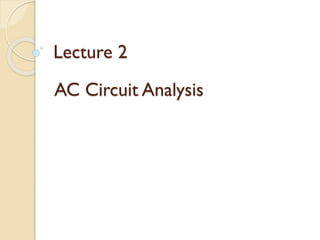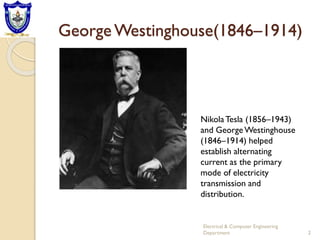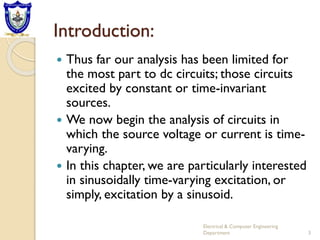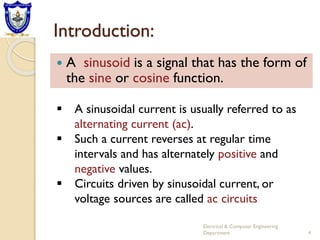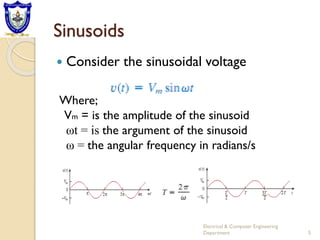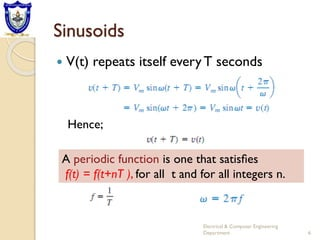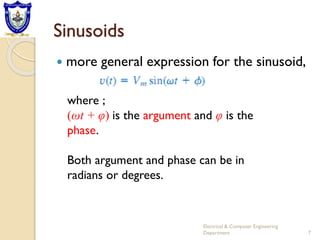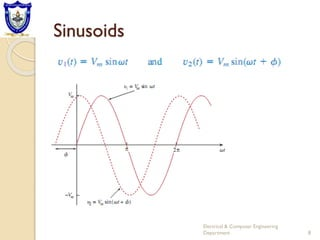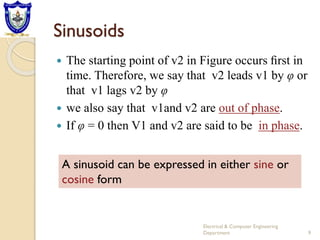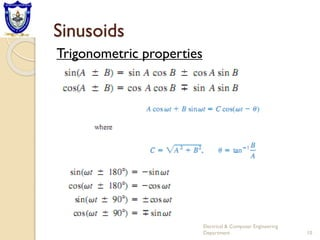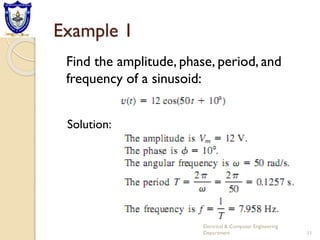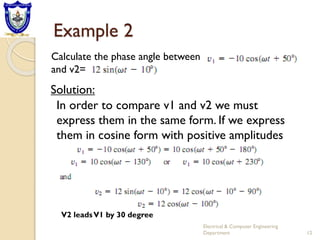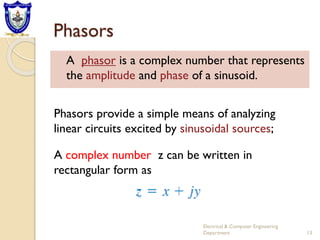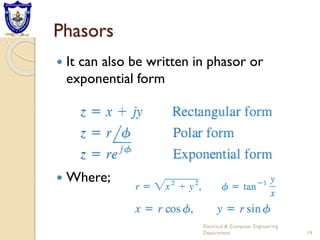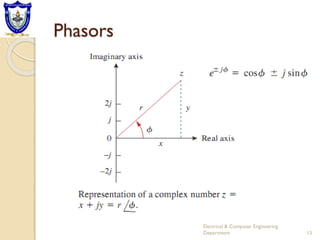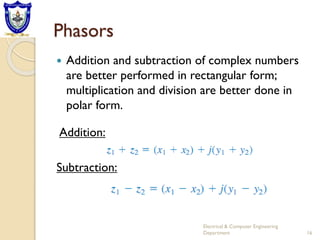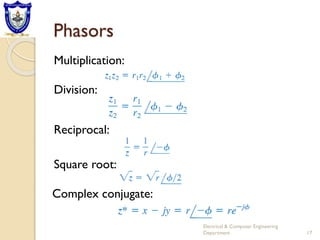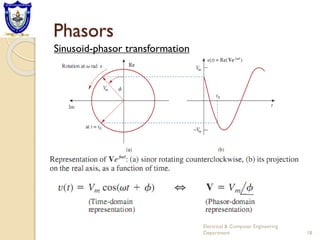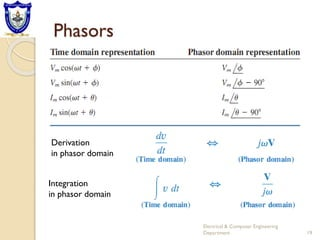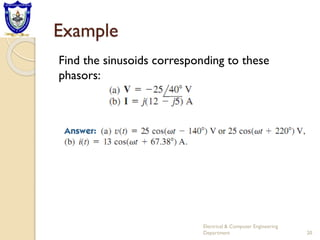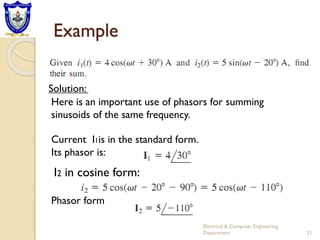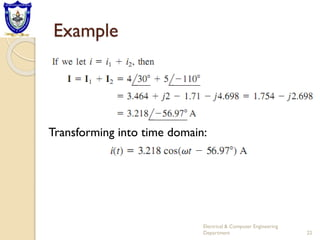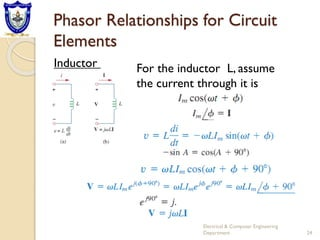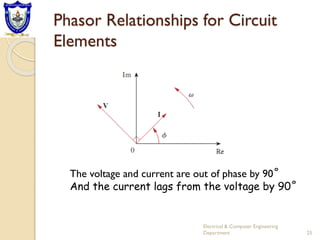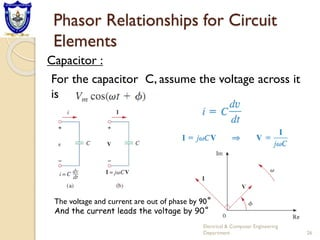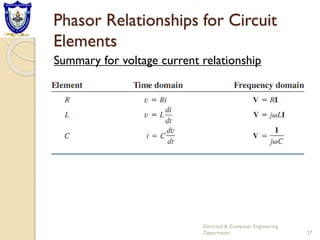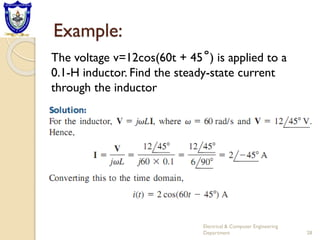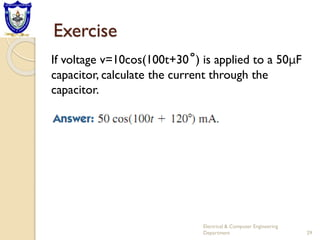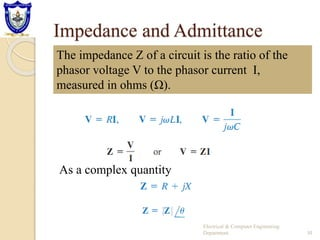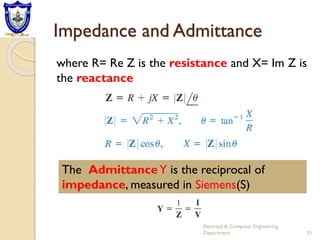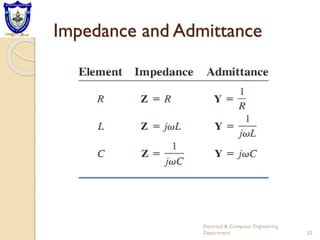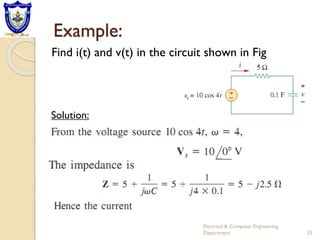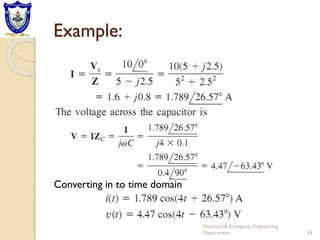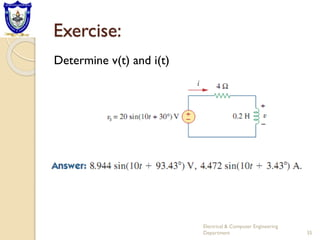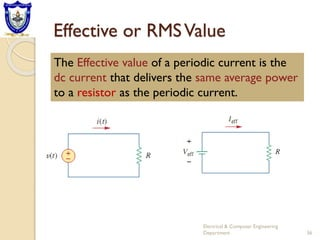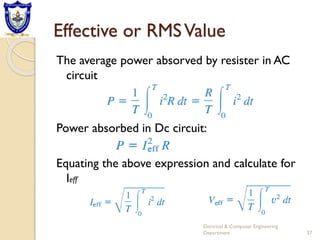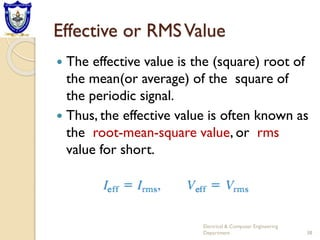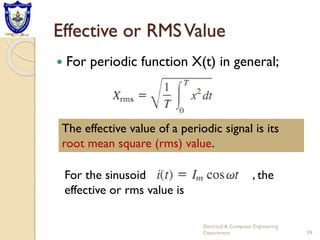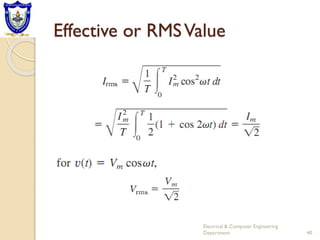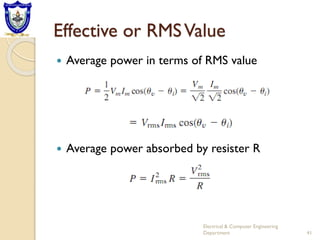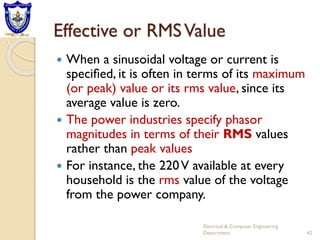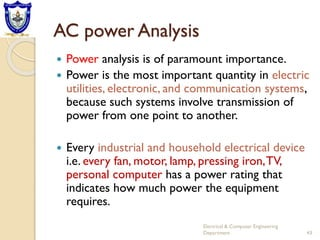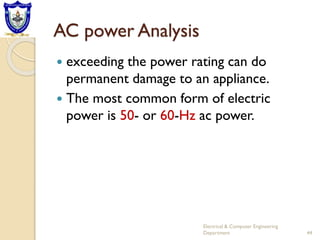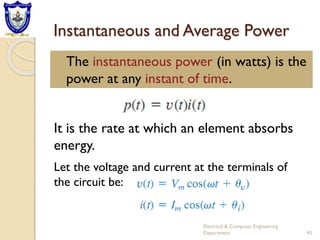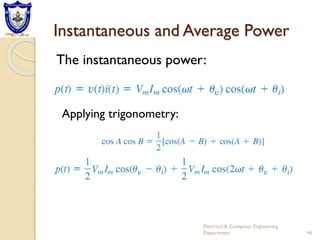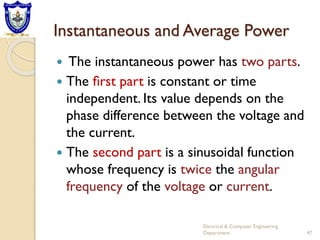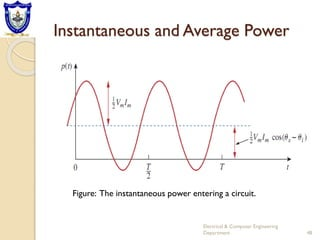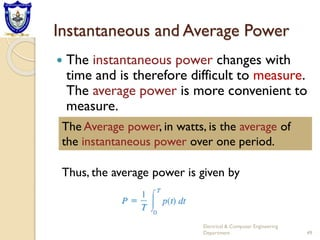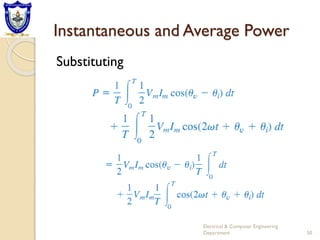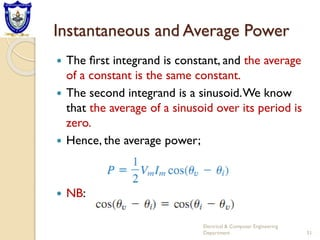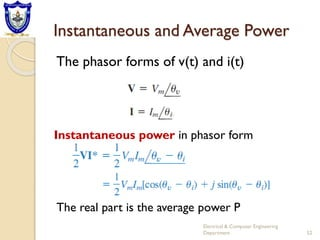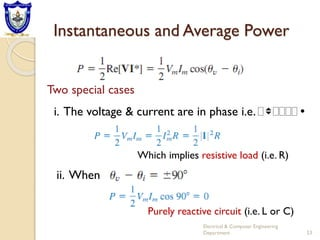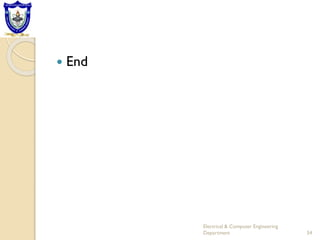1 sur 54

### Ch-2 AC.pdf

• 1. Lecture 2 AC Circuit Analysis
• 2. George Westinghouse(1846–1914) 2 Electrical & Computer Engineering Department NikolaTesla (1856–1943) and GeorgeWestinghouse (1846–1914) helped establish alternating current as the primary mode of electricity transmission and distribution.
• 3. Introduction:  Thus far our analysis has been limited for the most part to dc circuits; those circuits excited by constant or time-invariant sources.  We now begin the analysis of circuits in which the source voltage or current is time- varying.  In this chapter, we are particularly interested in sinusoidally time-varying excitation, or simply, excitation by a sinusoid. 3 Electrical & Computer Engineering Department
• 4. Introduction:  A sinusoid is a signal that has the form of the sine or cosine function.  A sinusoidal current is usually referred to as alternating current (ac).  Such a current reverses at regular time intervals and has alternately positive and negative values.  Circuits driven by sinusoidal current, or voltage sources are called ac circuits 4 Electrical & Computer Engineering Department
• 5. Sinusoids  Consider the sinusoidal voltage Where; Vm = is the amplitude of the sinusoid ωt = is the argument of the sinusoid ω = the angular frequency in radians/s 5 Electrical & Computer Engineering Department
• 6. Sinusoids  V(t) repeats itself everyT seconds Hence; A periodic function is one that satisﬁes f(t) = f(t+nT ), for all t and for all integers n. 6 Electrical & Computer Engineering Department
• 7. Sinusoids  more general expression for the sinusoid, where ; (ωt + φ) is the argument and φ is the phase. Both argument and phase can be in radians or degrees. 7 Electrical & Computer Engineering Department
• 8. Sinusoids 8 Electrical & Computer Engineering Department
• 9. Sinusoids  The starting point of v2 in Figure occurs ﬁrst in time. Therefore, we say that v2 leads v1 by φ or that v1 lags v2 by φ  we also say that v1and v2 are out of phase.  If φ = 0 then V1 and v2 are said to be in phase. A sinusoid can be expressed in either sine or cosine form 9 Electrical & Computer Engineering Department
• 10. Sinusoids 10 Electrical & Computer Engineering Department Trigonometric properties
• 11. Example 1 Find the amplitude, phase, period, and frequency of a sinusoid: Solution: 11 Electrical & Computer Engineering Department
• 12. Example 2 Calculate the phase angle between and v2= In order to compare v1 and v2 we must express them in the same form. If we express them in cosine form with positive amplitudes Solution: V2 leadsV1 by 30 degree 12 Electrical & Computer Engineering Department
• 13. Phasors A phasor is a complex number that represents the amplitude and phase of a sinusoid. Electrical & Computer Engineering Department 13 A complex number z can be written in rectangular form as Phasors provide a simple means of analyzing linear circuits excited by sinusoidal sources;
• 14. Phasors  It can also be written in phasor or exponential form  Where; Electrical & Computer Engineering Department 14
• 15. Phasors Electrical & Computer Engineering Department 15
• 16. Phasors  Addition and subtraction of complex numbers are better performed in rectangular form; multiplication and division are better done in polar form. Electrical & Computer Engineering Department 16 Addition: Subtraction:
• 17. Phasors Electrical & Computer Engineering Department 17 Multiplication: Division: Reciprocal: Square root: Complex conjugate:
• 18. Phasors Electrical & Computer Engineering Department 18 Sinusoid-phasor transformation
• 19. Phasors Electrical & Computer Engineering Department 19 Derivation in phasor domain Integration in phasor domain
• 20. Example Find the sinusoids corresponding to these phasors: Electrical & Computer Engineering Department 20 12j -j^2 5 but j^2= -1 i=5+12j
• 21. Example Electrical & Computer Engineering Department 21 Here is an important use of phasors for summing sinusoids of the same frequency. I2 in cosine form: Phasor form Solution: Current I1is in the standard form. Its phasor is:
• 22. Example Electrical & Computer Engineering Department 22 Transforming into time domain:
• 23. Phasor Relationships for Circuit Elements Electrical & Computer Engineering Department 23 Resister If the current through the resister is: The phasor form of the voltage
• 24. Phasor Relationships for Circuit Elements Electrical & Computer Engineering Department 24 Inductor For the inductor L, assume the current through it is
• 25. Phasor Relationships for Circuit Elements Electrical & Computer Engineering Department 25 The voltage and current are out of phase by 90˚ And the current lags from the voltage by 90˚
• 26. Phasor Relationships for Circuit Elements Electrical & Computer Engineering Department 26 Capacitor : For the capacitor C, assume the voltage across it is The voltage and current are out of phase by 90˚ And the current leads the voltage by 90˚
• 27. Phasor Relationships for Circuit Elements Electrical & Computer Engineering Department 27 Summary for voltage current relationship
• 28. Example: Electrical & Computer Engineering Department 28 The voltage v=12cos(60t + 45˚) is applied to a 0.1-H inductor. Find the steady-state current through the inductor
• 29. Exercise Electrical & Computer Engineering Department 29 If voltage v=10cos(100t+30˚) is applied to a 50μF capacitor, calculate the current through the capacitor.
• 30. Impedance and Admittance Electrical & Computer Engineering Department 30 The impedance Z of a circuit is the ratio of the phasor voltage V to the phasor current I, measured in ohms (Ω). As a complex quantity
• 31. Impedance and Admittance Electrical & Computer Engineering Department 31 where R= Re Z is the resistance and X= Im Z is the reactance The AdmittanceY is the reciprocal of impedance, measured in Siemens(S)
• 32. Impedance and Admittance Electrical & Computer Engineering Department 32
• 33. Example: Electrical & Computer Engineering Department 33 Find i(t) and v(t) in the circuit shown in Fig Solution:
• 34. Example: Electrical & Computer Engineering Department 34 Converting in to time domain
• 35. Exercise: Electrical & Computer Engineering Department 35 Determine v(t) and i(t)
• 36. Effective or RMSValue Electrical & Computer Engineering Department 36 The Effective value of a periodic current is the dc current that delivers the same average power to a resistor as the periodic current.
• 37. Effective or RMSValue The average power absorved by resister in AC circuit Power absorbed in Dc circuit: Equating the above expression and calculate for Ieff Electrical & Computer Engineering Department 37
• 38. Effective or RMSValue  The effective value is the (square) root of the mean(or average) of the square of the periodic signal.  Thus, the effective value is often known as the root-mean-square value, or rms value for short. Electrical & Computer Engineering Department 38
• 39. Effective or RMSValue  For periodic function X(t) in general; Electrical & Computer Engineering Department 39 The effective value of a periodic signal is its root mean square (rms) value. For the sinusoid , the effective or rms value is
• 40. Effective or RMSValue Electrical & Computer Engineering Department 40
• 41. Effective or RMSValue  Average power in terms of RMS value  Average power absorbed by resister R Electrical & Computer Engineering Department 41
• 42. Effective or RMSValue  When a sinusoidal voltage or current is speciﬁed, it is often in terms of its maximum (or peak) value or its rms value, since its average value is zero.  The power industries specify phasor magnitudes in terms of their RMS values rather than peak values  For instance, the 220V available at every household is the rms value of the voltage from the power company. Electrical & Computer Engineering Department 42
• 43. AC power Analysis  Power analysis is of paramount importance.  Power is the most important quantity in electric utilities, electronic, and communication systems, because such systems involve transmission of power from one point to another.  Every industrial and household electrical device i.e. every fan, motor, lamp, pressing iron,TV, personal computer has a power rating that indicates how much power the equipment requires. Electrical & Computer Engineering Department 43
• 44. AC power Analysis  exceeding the power rating can do permanent damage to an appliance.  The most common form of electric power is 50- or 60-Hz ac power. Electrical & Computer Engineering Department 44
• 45. Instantaneous and Average Power The instantaneous power (in watts) is the power at any instant of time. Electrical & Computer Engineering Department 45 It is the rate at which an element absorbs energy. Let the voltage and current at the terminals of the circuit be:
• 46. Instantaneous and Average Power The instantaneous power: Electrical & Computer Engineering Department 46 Applying trigonometry:
• 47. Instantaneous and Average Power  The instantaneous power has two parts.  The ﬁrst part is constant or time independent. Its value depends on the phase difference between the voltage and the current.  The second part is a sinusoidal function whose frequency is twice the angular frequency of the voltage or current. Electrical & Computer Engineering Department 47
• 48. Instantaneous and Average Power Electrical & Computer Engineering Department 48 Figure: The instantaneous power entering a circuit.
• 49. Instantaneous and Average Power  The instantaneous power changes with time and is therefore difficult to measure. The average power is more convenient to measure. Electrical & Computer Engineering Department 49 The Average power, in watts, is the average of the instantaneous power over one period. Thus, the average power is given by
• 50. Instantaneous and Average Power Substituting Electrical & Computer Engineering Department 50
• 51. Instantaneous and Average Power  The ﬁrst integrand is constant, and the average of a constant is the same constant.  The second integrand is a sinusoid.We know that the average of a sinusoid over its period is zero.  Hence, the average power;  NB: Electrical & Computer Engineering Department 51
• 52. Instantaneous and Average Power The phasor forms of v(t) and i(t) Electrical & Computer Engineering Department 52 The real part is the average power P Instantaneous power in phasor form
• 53. Instantaneous and Average Power Electrical & Computer Engineering Department 53 Two special cases i. The voltage & current are in phase i.e. vi Which implies resistive load (i.e. R) ii. When Purely reactive circuit (i.e. L or C)
• 54.  End Electrical & Computer Engineering Department 54